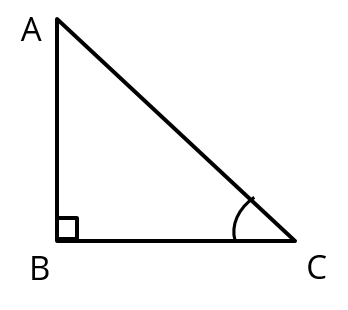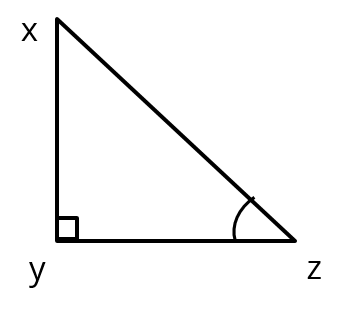# Trigonometry Extra Questions | Class 10 Maths | Q3

###### NCERT Chapter 8 | Trigonometric Values & Pythagoras Theorem | Similar TrianglesClass 10 Math Questions

This CBSE class 10 Maths additional practice question is from the topic Trigonometry. Concepts Covered: Trigonometric Values, Pythagoras Theorem and Similarity of Triangles.

Question 3 : If C and Z are acute angles and that cos C = cos Z prove that ∠C = ∠Z .

## NCERT Solution to Class 10 Maths

### Explanatory Answer | Trigonometry Important Question 3

#### Let us assume two right triangles ΔABC and ΔXYZGiven C and Z are acute angles and cos C = cos Z
cos C = $$frac{\text{BC}}{\text{AC}}$ and cos Z = $\frac{\text{YZ}}{\text{XZ}}$ cos C = cos Z =>$\frac{\text{BC}}{\text{AC}}$= $\frac{\text{YZ}}{\text{XZ}}$ Let $\frac{\text{BC}}{\text{YZ}}$= $\frac{\text{AC}}{\text{XZ}}$ = k.....$1)
BC = k(yz) and AC = k(xz)

In right triangle ABC, using Pythagoras theorem AB = $$sqrt{AC^2− BC^2 }$ AB = $\sqrt{$k(xz$^2−(k(yz)^2)})= k$$sqrt{$xz$^2− (yz)^2 })
In right triangle xyz, using Pythagoras theorem xy = $$sqrt{$xz$^2− (yz)^2 })
$$frac{\text{AB}}{\text{XY}}$ = $\frac{K × \sqrt{$X Z$^{2}-(Y Z)^{2}}}{$sqrt{$X Z)^{2}-(Y Z)^{2}}})
Or $$frac{\text{AB}}{\text{XY}}$ = k .....$1)

From 1 and 2, $\frac{\text{BC}}{\text{YZ}}$ = $\frac{\text{AC}}{\text{XZ}}$ = $\frac{\text{AB}}{\text{XY}}$ = k
By SSS similarity criterion, we can conclude ΔABC is similar to ΔXYZ
∴ Corresponding angles of two similar triangles will be equal.
∠C = ∠Z

###### Free CBSE Online CoachingClass 10 Maths

Register in 2 easy steps and
Start learning in 5 minutes!